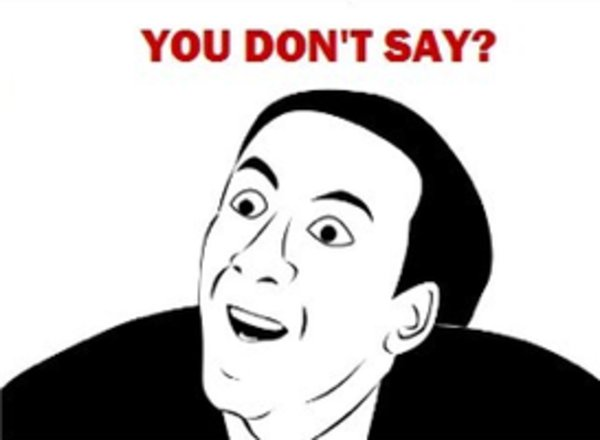Dear All,

I have two questions about DCF. The first is that most of the time when you do a DCF, you start with EBIT*(1-tax) and then +depreciation-capex+/- change in working capital to arrive at free cash flow to firm. And the tax is certainly no the tax expense in income statement.

However in the following extract from an analyst report, when doing a DCF analysis, the analyst start with EBIT and then minus the tax expense in the income statement instead of using the EBIT*(1-tax) formula, is he correct in doing this(you can look at the number in the tax expense in income statement and that is the same as the tax payment in DCF)?

Second questions is that usually the income from investment in associate is not included in the calculation of free cash flow to firm but investment in associate is treated as non core asset and subtract from the enterprise value. however in the following extract, the analyst include the income from investment in associate in the calculation of free cashflow and do not subtract the investment in associate from the enterprise value, , is he correct in doing this?

the income statement, balance sheet, cashflow statement and DCF is in below, appreciate any comment or help, thank you

income statement

balance sheet

cashflow statement

DCF

EBIT × (1 – tax rate) = (EBIT × 1) – (EBIT × tax rate) = EBIT – tax expense

hello S2000magician,

thank you for your help, i think this is not the first time i ask a question and you kindly response.

my question is if your EBIT is \$100, interest is \$20 and tax rate is 30%, then the tax expense in income statement is (100-20)*30%=24

and if we are using the EBIT*(1-tax rate), then the tax expense is 100*30%=30, so there is a difference in tax

and so i think it is wrong to use the tax expense directly from the income statement in doing a DCF

but that’s what the analyst do, he just use the tax expense in the income statement instead of using the EBIT*(1-tax rate) and so i wonder is it correct or not?

And what about his treatment of including the income from associate in calculating the DCF, is it legitimate to do this ?

Thank you very much again

Hi bruv,

Altough I passed Level III and am quite smart I had to google it again. Basically DCF works with free-cash-flow to the firm; meaning that you’re not looking at debt servicing.

It’s like “how much cash does the firm generate on a year, regardless of how it is financed ?” You’re trying to assess the value of its business model.

You would involve interest payments when calculating FCF to equity for example.

FCF to equity answers to another question “how much cash can the firm distribute to me, being an equity owner?”

You have a lot of better and clearer pages available on the internet, but basically you need to understand the difference between FCF and FCF to equity to answer your question